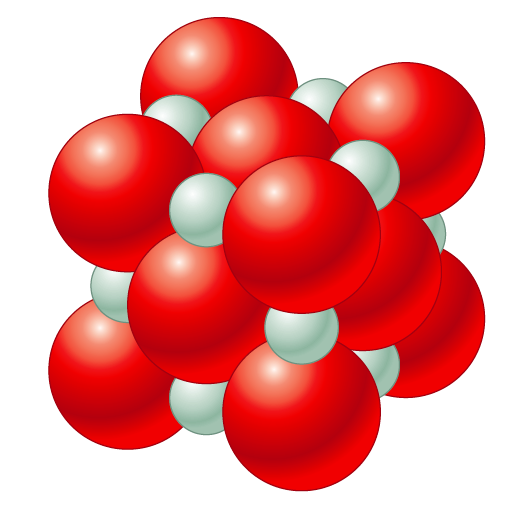# Problem: The unit cell of MgO is shown below.Does MgO have a structure like that of NaCl or ZnS? If the density of MgO is 3.58 g/cm3, estimate the radius (in centimeters) of the O2- anions and the Mg2+ cations.

###### FREE Expert Solution

NaCl  face-centered cubic (FCC)  lattice

• contains an atom in each of the face of the cube and an atom in each of the corners

ZnS  body-centered cubic (BCC) unit cell

•  composed of a cube with one atom at each of its corners and one atom at the center of the cube.

MgO:

• Has one O2- ion:
• at each corner
• at each face

MgO follows an FCC cubic lattice, similar to NaCl structure.

# of atoms = 4 per 1 unit cell

Step 1: Solve for volume using density:

volume = 7.477 x 10-23 cm3/1 unit cell

83% (81 ratings)###### Problem Details

The unit cell of MgO is shown below.Does MgO have a structure like that of NaCl or ZnS? If the density of MgO is 3.58 g/cm3, estimate the radius (in centimeters) of the O2- anions and the Mg2+ cations.